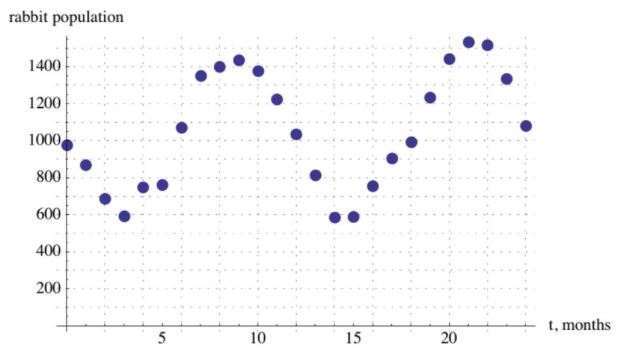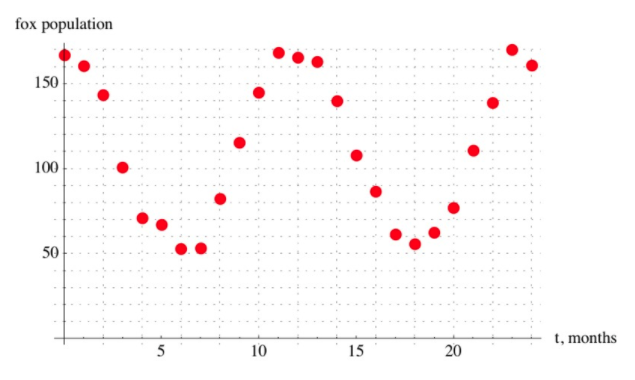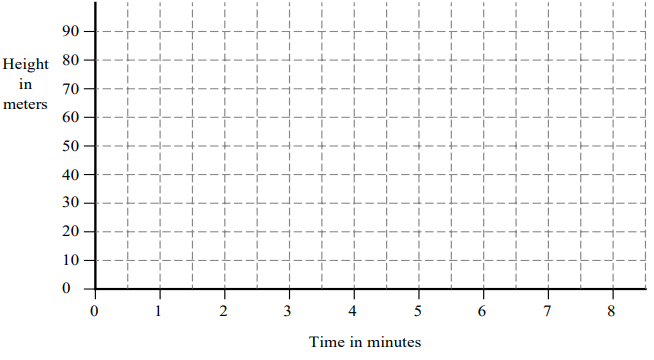# Unit Circle and Trigonometric Functions

## Objective

Model contextual situations using trigonometric functions (Part I).

## Common Core Standards

### Core Standards

?

• F.TF.A.3 — Use special triangles to determine geometrically the values of sine, cosine, tangent for π/3, π/4 and π/6, and use the unit circle to express the values of sine, cosine, and tangent for π-x, π+x, and 2π-x in terms of their values for x, where x is any real number.

• F.TF.B.5 — Choose trigonometric functions to model periodic phenomena with specified amplitude, frequency, and midline. Modeling is best interpreted not as a collection of isolated topics but in relation to other standards. Making mathematical models is a Standard for Mathematical Practice, and specific modeling standards appear throughout the high school standards indicated by a star symbol (★). The star symbol sometimes appears on the heading for a group of standards; in that case, it should be understood to apply to all standards in that group.

## Criteria for Success

?

1. Describe the meaning of parameters for sine and cosine functions in context.
2. Verify and describe the model in the context of the situation.
3. Define alternative models that may be used for a contextual situation.

## Anchor Problems

?

### Problem 1

Given below are two graphs that show the populations of foxes and rabbits in a national park over a 24 month period.a. Explain why it is appropriate to model the number of rabbits and foxes as trigonometric functions of time.

b. Find an appropriate trigonometric function that models the number of rabbits, ${r(t)}$, as a function of time, with $t$ in months.

c. FInd an appropriate trigonometric function that models the number of foxes, $f(t)$, as a function of time, with $t$ in months.

#### References

Illustrative Mathematics Foxes and Rabbits 3

Foxes and Rabbits 3, accessed on Feb. 26, 2018, 4:39 p.m., is licensed by Illustrative Mathematics under either the CC BY 4.0 or CC BY-NC-SA 4.0. For further information, contact Illustrative Mathematics.

### Problem 2

Have students participate in the Matching Graphs and Functions activity on page T-6 in Representing Trigonometric Functions from MARS.

#### References

MARS Formative Assessment Lessons for High School Representing Trigonometric Functions

Representing Trigonometric Functions from the Classroom Challenges by the MARS Shell Center team at the University of Nottingham is made available by the Mathematics Assessment Project under the CC BY-NC-ND 3.0 license. Copyright © 2007-2015 Mathematics Assessment Resource Service, University of Nottingham. Accessed Feb. 26, 2018, 4:40 p.m..

Modified by Fishtank Learning, Inc.

## Problem Set

?

The following resources include problems and activities aligned to the objective of the lesson that can be used to create your own problem set.

?

A Ferris wheel is 50 meters in diameter and rotates once every three minutes. The center axle of the Ferris wheel is 30 meters from the ground.

a. Using the axes below, sketch a graph to show how the height of a passenger will vary with time. Assume that when the wheel starts rotating when the passenger is at the bottom.b. A mathematical model for this motion is given by the formula

${h=a+b\mathrm{cos}ct}$    where     $h$= the height of the car in meters

$t$= the time that has elapsed in minutes

${a, b, c}$ are constants

Find values for $a$$b$, and $c$ that will model this situation.